## What is Read Only Memory (ROM) & Random Access Memory (RAM)?# What is Read Only Memory (ROM) & Random Access Memory (RAM)?

As the amount of information increases, We need to design circuits to help us abstract the increased information so that we can successfully design a larger system.

There are primarily two types of circuits that you can use to store and retrieve large amounts of information.

2. Random Access Memory or RAMs.

Before I describe how to design these storage devices, I want to explain the principles that we will use through an analogy. Suppose that you want to store information about all the homes in a large city, in which rooms if televisions in them. Were the sake of simplicity we’ll assume that every house has the same number of rooms. We’ll need to know where to find the houses and then we can identify which rooms have televisions. Just like the way we use numbers in streets to address a house, We could encode the information about where to find the house as a binary address.

In this example, we have four houses so we need only two bits to encode the location of each house.. So our the city can be described by a set of address bits, and a set of data bits.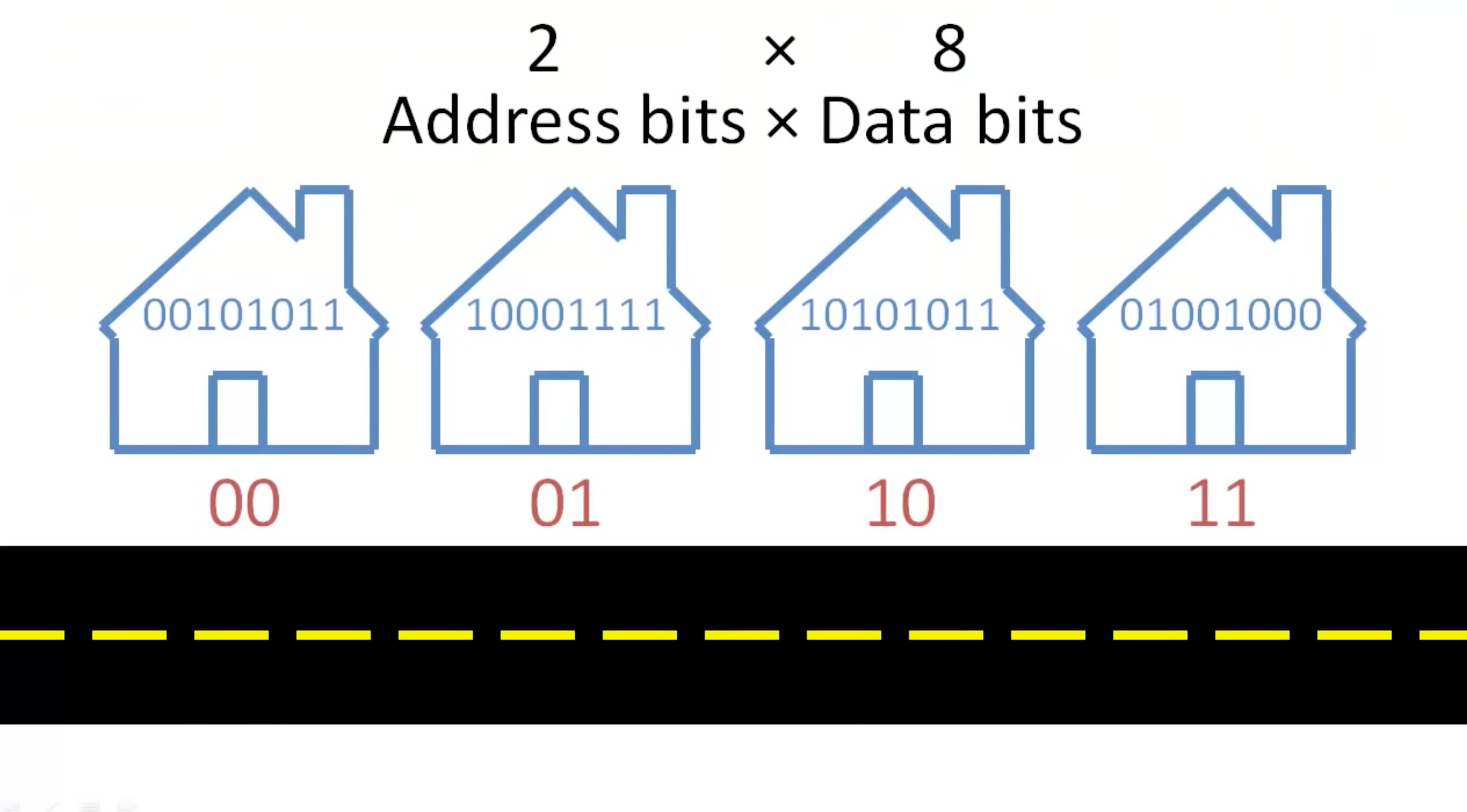If we increase the number of houses we need to increase the number of addresses, and each additional address bit doubles the number of addresses that we can encode.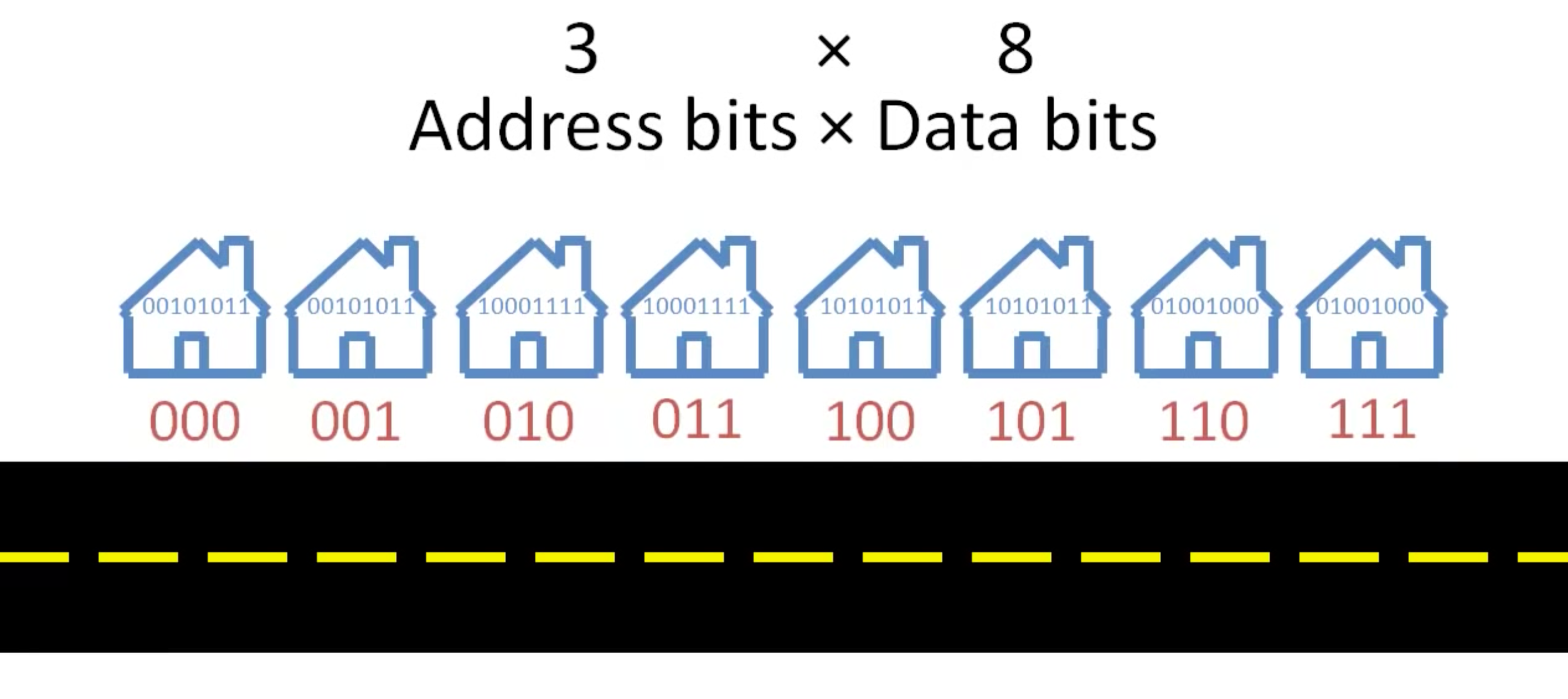If we increase the number of rooms in each house we will need to add one bit for each room. Notice, that adding one data bit does not double the number of rooms that we can describe because each data bit describes the data. Address bits encode information about the location, but data bits are the information.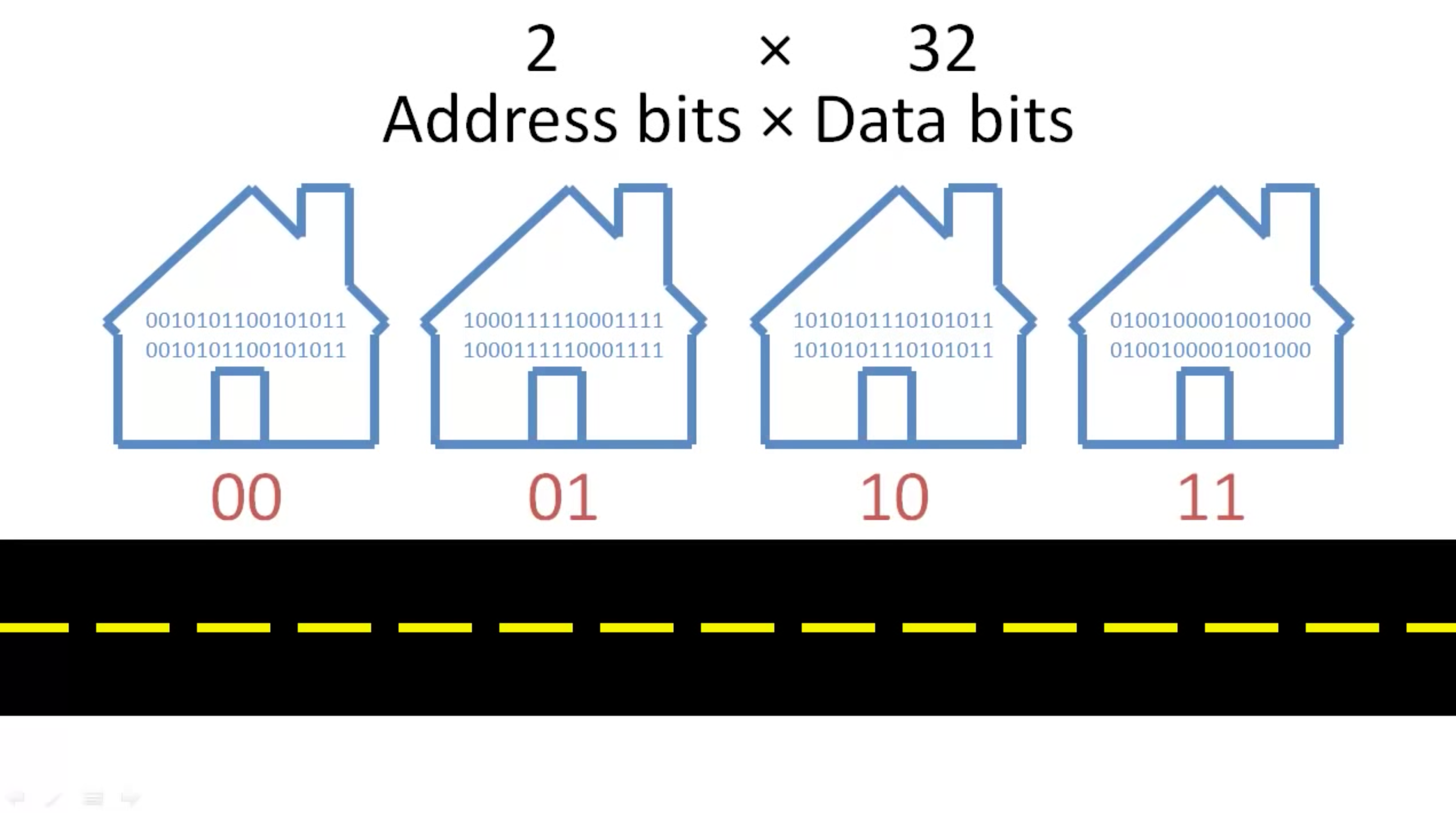Similarly, when we design ROMs and RAMs, we use two different types of bits. First, we encode where to find specific information within a set of address bits. These addresses indicate words that all contain the same number of bits. These data bits are information much like whether each room in the house is the television. The overall storage capacity of a ROM or RAM can be calculated by multiplying the number of addresses by the number of bits starts at each address.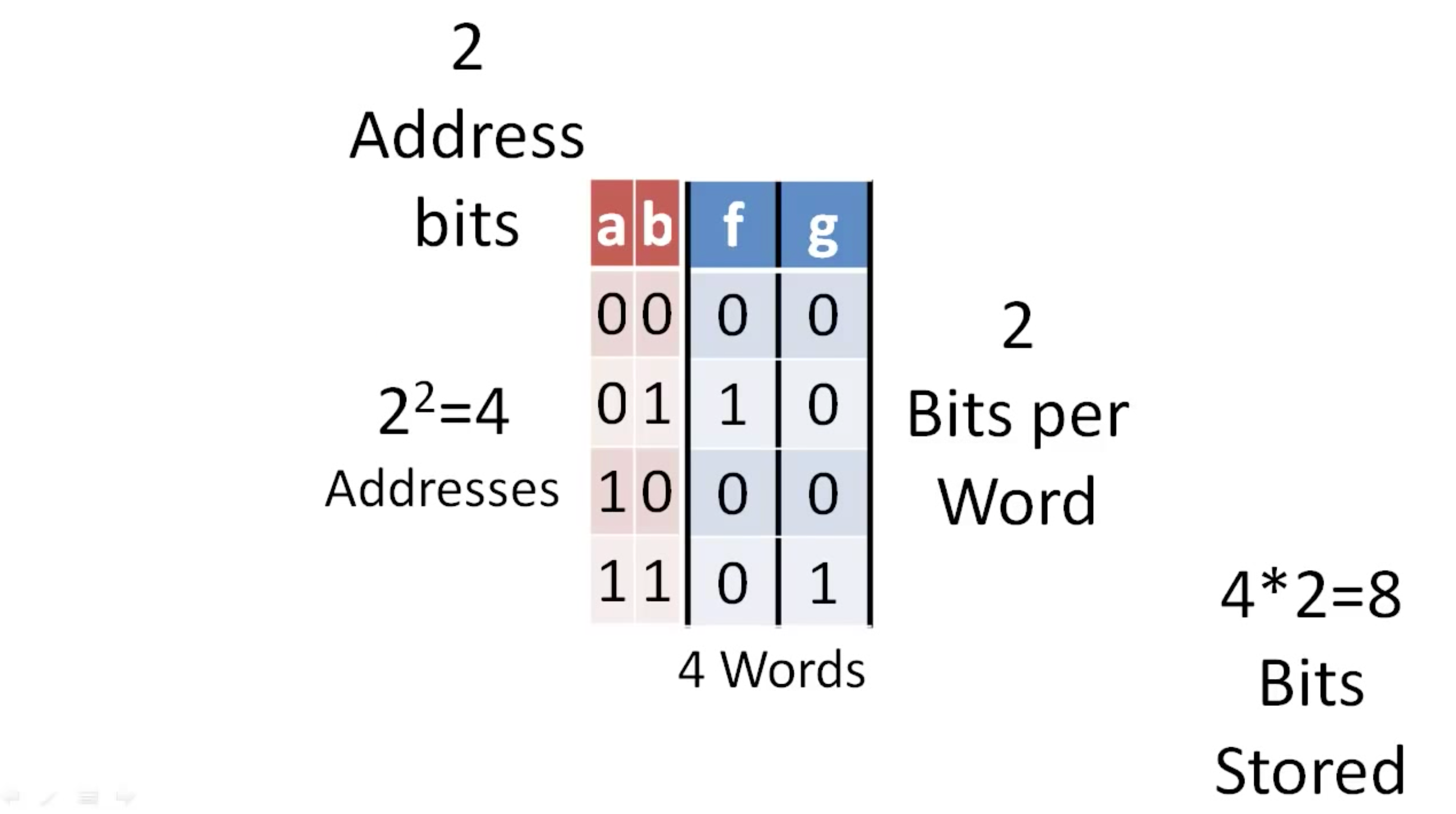If we want to double the number of addresses in a storage device we simply need to add one additional address bit. In contrast, if we want to double the number of bits per word, we need to double the number of bits stored at each address.

When we include a storage device in a circuit, we will typically indicate the dimensions of the storage device where the first number reflects the number of addresses and the second number reflects the number of data bits per word. Although, ROMs and RAMs are both information storage devices that store information at addresses in words of a fixed length, they serve different purposes in a computer.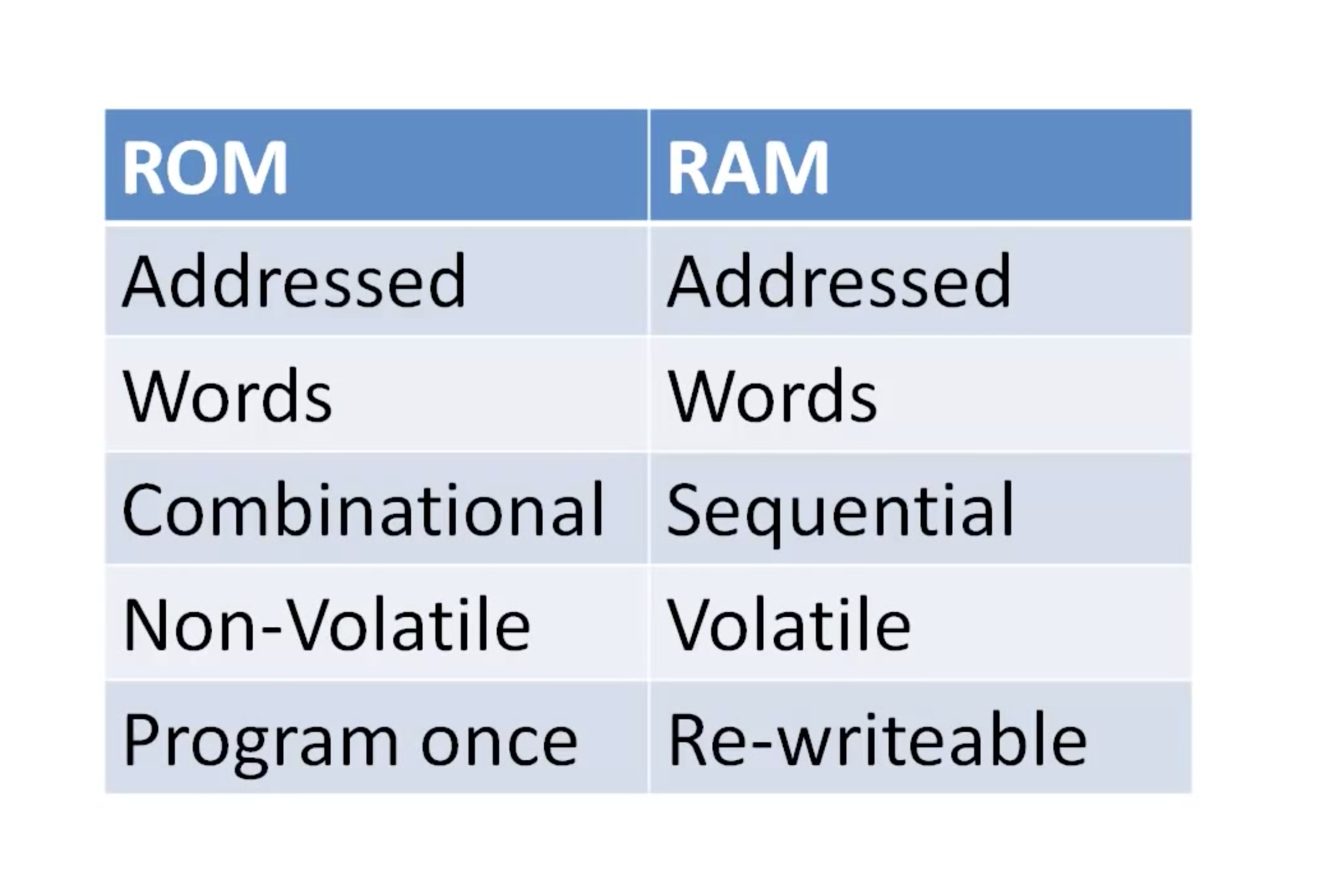ROMs are devices that are combinational circuits that our the program wants ideally, and will never lose their information even more ideally, even when they lose power. In contrast, RAMs are volatile and lose information, they lose power but they can be easily rewritten because they are based on sequential circuits.

Latest Posts

##### Effective Debugging Techniques in VHDL: Mastering the Art of Troubleshooting

Hot items

• 0.00

###### Resume Templates Mega Bundle For Electronics Engineers